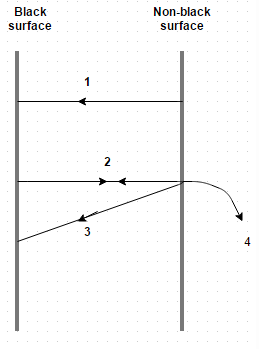Courses

# Test: Kirchoff’s Law

## 10 Questions MCQ Test Heat Transfer | Test: Kirchoff’s Law

Description
This mock test of Test: Kirchoff’s Law for Chemical Engineering helps you for every Chemical Engineering entrance exam. This contains 10 Multiple Choice Questions for Chemical Engineering Test: Kirchoff’s Law (mcq) to study with solutions a complete question bank. The solved questions answers in this Test: Kirchoff’s Law quiz give you a good mix of easy questions and tough questions. Chemical Engineering students definitely take this Test: Kirchoff’s Law exercise for a better result in the exam. You can find other Test: Kirchoff’s Law extra questions, long questions & short questions for Chemical Engineering on EduRev as well by searching above.
QUESTION: 1

### If radiant energy EB emitted by the black surface strikes the non-black surface. If non-black surface has absorptivity α, it will absorb how much radiations?

Solution:

The remainder (1 – α) will be reflected back for full absorption at the black surface.

QUESTION: 2

### If two surfaces are at the same temperature, then the conditions correspond to mobile thermal equilibrium for which the resultant interchange of heat is zero are

Solution:

The remainder (1 – α) will be reflected back for full absorption at the black surface.

QUESTION: 3

### The absorptivity of black body equals to

Solution:

The absorptivity of black body equals to unity.

QUESTION: 4

A diathermanous body

Solution:

It behaves like a body that allows all the incident radiation to pass through it.

QUESTION: 5

Choose the false statement

Solution:

Rough surfaces give diffused reflections. Reflections from highly polished and smooth surfaces have regular characteristics.

QUESTION: 6

The emissivity and the absorptivity of a real surface are equal for radiation with identical temperature and wavelength. This law is referred to as

Solution:

Emissivity and absorptivity are related by Kirchhoff’s law.

QUESTION: 7

With an increase in wavelength, the monochromatic emissive power of a black body

Solution:

It firstly increases to its maximum value and then decreases to zero.

QUESTION: 8

The temperature of a solid surface changes from 27 K to 627 K. The emissive power changes would then confirm to the ratio

Solution:

E2/E1 = (T2/T1)4 = 81.

QUESTION: 9

If the temperature of a hot body is increased by 50%, the amount of radiations emitted by it would increase by nearly

Solution:

E2/E1 = (T2/T1)4 = 5.06.

QUESTION: 10

Consider two surfaces, one absolutely black and the other non-black. These surfaces are arranged parallel to each other and so close that the radiation of one falls totally on the other. Choose the correct optionSolution:

The radiant energy E emitted by the black surface strikes the non-black surface. If the non-black surface has absorptivity α, it will absorb α E b radiations.